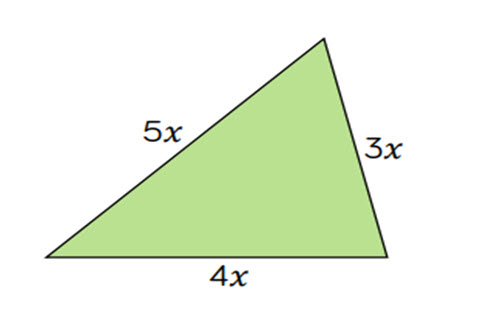# Algebra: finding rules, forming expressions and solving equations

This guide provides a sequence of small steps for working algebraically, from exploring one-step and two-step function machines, using substitution and formulae, through to forming and solving equations.

Year level(s) Year 6, Year 5
Audience Teacher
Purpose Teaching resource, Teaching strategies
Teaching strategies and pedagogical approaches Questioning, Explicit teaching
Keywords function, rule, reasoning, problem-solving, word problem, explicit teaching

## Curriculum alignment

Curriculum connections Numeracy
Strand and focus Algebra, Build understanding
Topics Algebraic expressions
AC: Mathematics (V9.0) content descriptions
AC9M5A02
Find unknown values in numerical equations involving multiplication and division using the properties of numbers and operations

AC9M6A01
Recognise and use rules that generate visually growing patterns and number patterns involving rational numbers

Numeracy progression Multiplicative strategies (P7)
Number patterns and algebraic thinking (P5)